# Translate words into number codes

In this worksheet, students will turn words into number codes using a given code table as a base. It will develop their encoding and problem solving skills.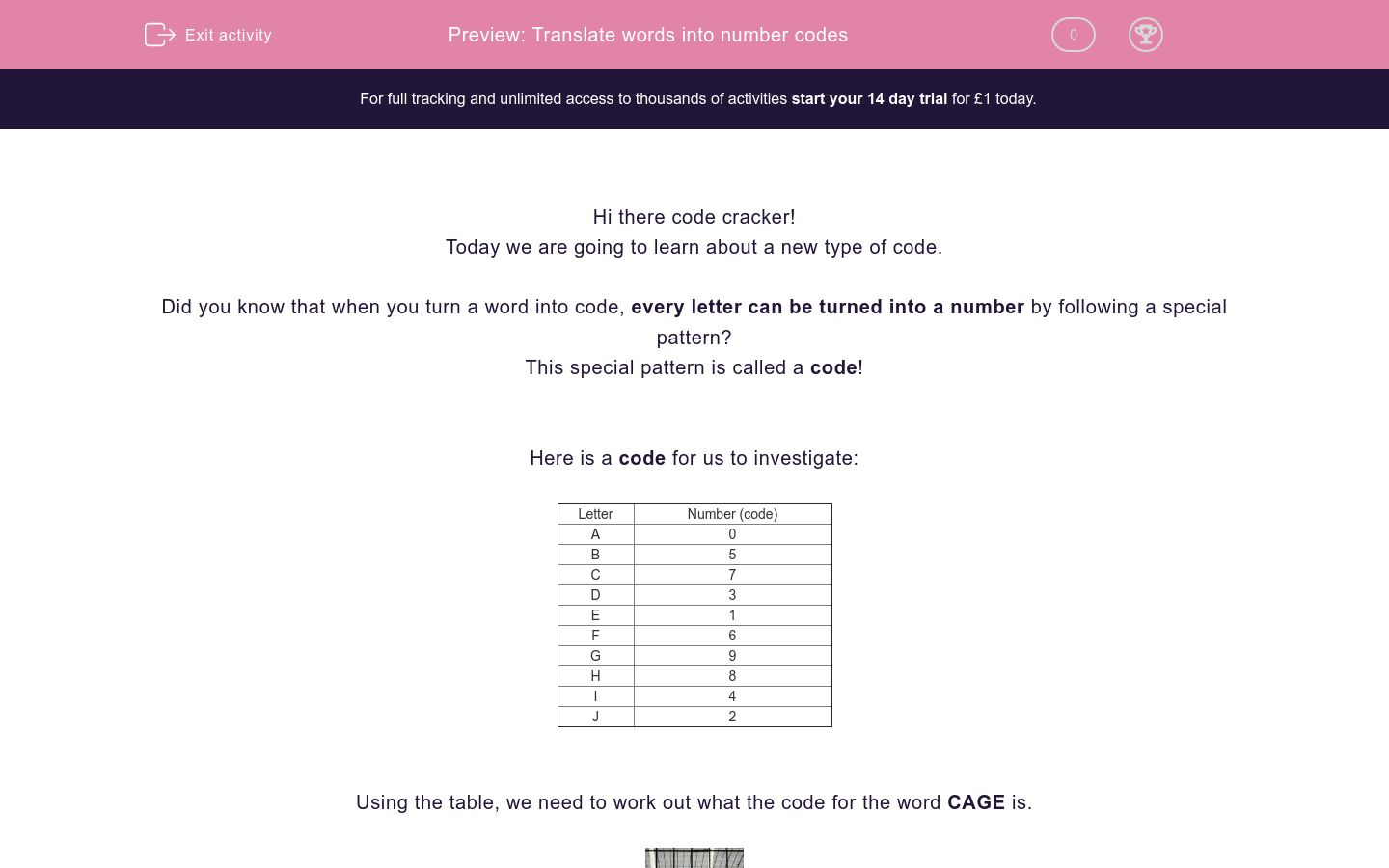Key stage:  KS 2

Curriculum topic:   Verbal Reasoning

Curriculum subtopic:   Number Code Breaking

Difficulty level:### QUESTION 1 of 10

Hi there code cracker!

Today we are going to learn about a new type of code.

Did you know that when you turn a word into code, every letter can be turned into a number by following a special pattern?

This special pattern is called a code!

Here is a code for us to investigate:

 Letter Number (code) A 0 B 5 C 7 D 3 E 1 F 6 G 9 H 8 I 4 J 2

Using the table, we need to work out what the code for the word CAGE is.If we start with finding the code for C and then work through each letter one-by-one, we find that the code for CAGE is 7091.

Now let’s reverse what we did to find out which word is represented by the code 8103.

Then we can repeat this process for each of the numbers one-by-one to find that the word is HEAD.

Is that what you found?

It’s now your turn to be a code cracker and change words into and out of code. Make sure you check the code table for each question.

Good luck code cracker!

 A = 2 B = 6 C = 4 E - 7 F = 9 K = 3 N = 1 R = 8

Right then, code cracker, let's get going with some number code breaking!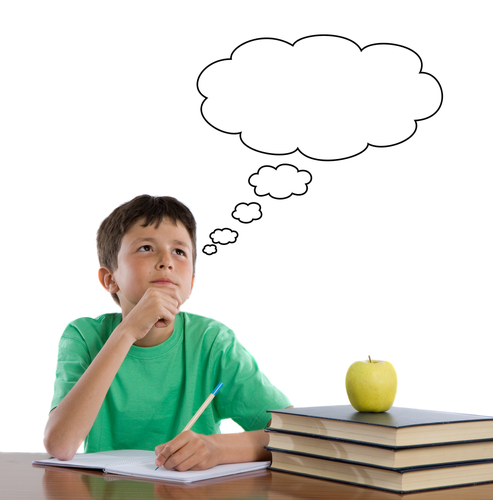So, in this worksheet we are going to try and make sure you learn the basic skills you need to become a number code breaking superstar. Using the code above to help you, can you work out the codes for the following words?

1. Far

2. Car

3. Race

4. Bank

## Column B

Far
928
Car
428
Race
8247
Bank
6213
 A = 5 E = 8 G = 9 I = 2 M = 6 N = 3 O = 7

So now that you have had a go at your first question, code cracker, I think you're ready to take on the challenge again! You can definitely do it!Just as in the previous question, you will need to use the code above to help you work out the number codes for the following words:

1. Gem

2. Gain

3. Main

4. Name

Can you match the correct code to the words below?

## Column B

Gem
6523
Gain
986
Main
9523
Name
3568
 C = 8 G = 4 H = 6 I = 1 K = 2 N = 7 O = 9 T = 5 U = 3

Let's keep going, code cracker! I know you are going to totally master the skill of number code breaking by the end of this worksheet and you will feel so proud of yourself!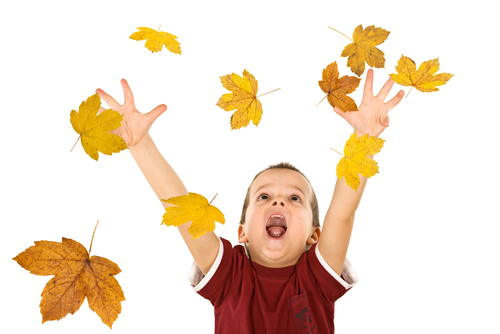So, as usual we need to use the table at the top to help us. Be really careful with this - it is your most important tool! Can you work out the codes for the words below and match the correct code to the correct word? I think you can do it!

1. Night

2. Think

3. Hug

4. Thought

5. Cough

## Column B

Night
634
Think
71465
Hug
89346
Thought
56172
Cough
5693465
 A = 6 E = 3 N = 2 O = 9 S = 8 W = 1 Y = 7

Ok, code cracker, I think you are ready for a new challenge! You are still going to be working out the number codes for different words using the table above, but now I am going to ask you to write them out yourself (instead of matching them) when you answer the question! Can you do it? Yes you can!Can you work out the number code for the following words?

1. Say

2. Yes

3. Soya

4. Yawn

Write out the codes in the correct boxes below.

## Column B

Night
634
Think
71465
Hug
89346
Thought
56172
Cough
5693465
 A = 7 C = 4 D = 3 E = 9 O = 2 R = 6 T = 1 U = 5

Let's keep going, code cracker, you're on a roll!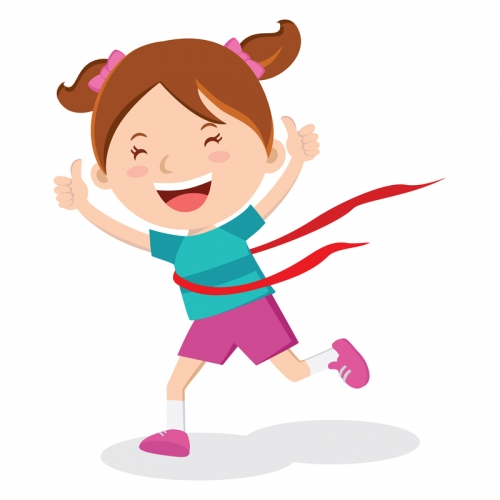Using the table, as usual, can you work out and write down the codes for the following words?

2. Doctor

3. Tear

4. Odour

## Column B

Night
634
Think
71465
Hug
89346
Thought
56172
Cough
5693465
 D = 7 F = 2 G = 4 H = 8 N = 6 O = 1 R = 3 T = 9 Y = 5 U = 0

Well done, code cracker, you've made it to another question! I think you'll like this one. It involves doughnuts...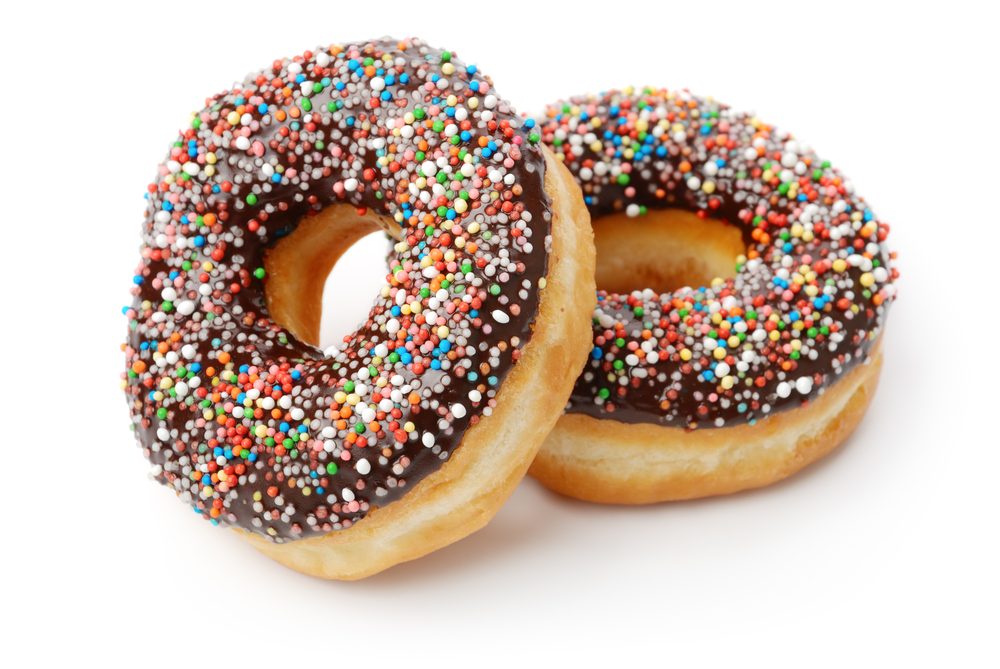I can tell you're interested in this one now! Right, let's get code cracking. What are the codes for the following words?

1. Four

2. Roof

3. Found

4. Doughnut

## Column B

Night
634
Think
71465
Hug
89346
Thought
56172
Cough
5693465
 A = 9 E = 5 I = 6 L = 1 P = 4 R = 7 T = 3

You are doing brilliantly, well done! You are definitely on your way to becoming a number code breaking medal winner!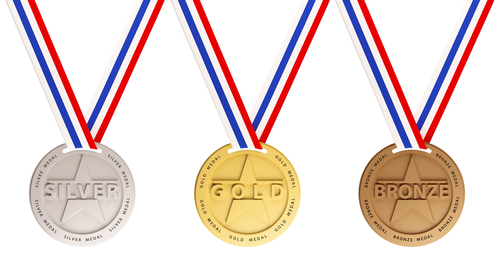So, now we are going to match the words and codes again as we are using some ever so slightly tricker words this time. Don't panic! You've got this! Can you match the correct codes to the correct words if the words are:

1. Reptile

2. Elite

3. Repeat

4. Tape

 7543615 3945 51635 754593 Reptile Elite Repeat Tape
 E = 7 G = 9 H = 4 I = 1 N = 5 O = 6 S = 2 T = 8 U = 3

We aren't too far away from the end, code cracker. Keep going!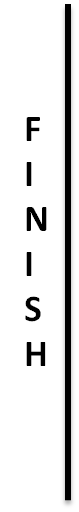So, you are going to do some matching again this time! Can you match the correct code to the correct word?!

1. Genius

2. Song

3. Singing

4. Ghost

 2659 2159159 975132 94628 Genius Song Singing Ghost
 D = 7 I = 1 K = 4 L = 9 N = 3 R = 2 S = 6

OK, then, code cracker! This is your second last question!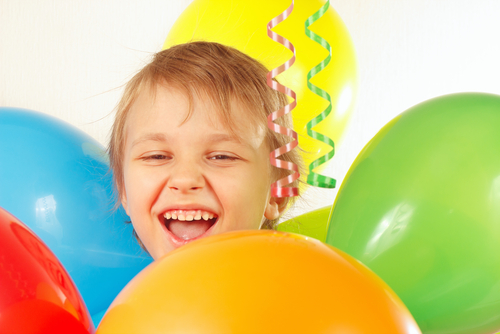So, can you work out and write down the codes for the following words:

1. Kind

2. Drink

3. Skin

Write out the codes below, leaving only one space between each code or else it will be marked as incorrect!

 2659 2159159 975132 94628 Genius Song Singing Ghost
 A = 3 B = 9 D = 4 E = 1 F = 6 L = 5 N = 2 O = 8 R = 7

You've made it, code cracker! What a fantastic effort, well done!Now, you just need to do one final thing: work out and write down the codes for the words below!

1. Before

2. Balloon

3. Fallen

4. Baboon

Write out the codes in the correct boxes below.

 2659 2159159 975132 94628 Genius Song Singing Ghost
• Question 1
 A = 2 B = 6 C = 4 E - 7 F = 9 K = 3 N = 1 R = 8

Right then, code cracker, let's get going with some number code breaking!So, in this worksheet we are going to try and make sure you learn the basic skills you need to become a number code breaking superstar. Using the code above to help you, can you work out the codes for the following words?

1. Far

2. Car

3. Race

4. Bank

## Column B

Far
928
Car
428
Race
8247
Bank
6213
EDDIE SAYS
How did you get on with this first test, code cracker? If you got it right, then give yourself a big pat on the back! Don't panic if you didn't get it right this time, though, it's only your first go after all. Let me show you how we get to the correct answers: To work out the code for the word FAR, we first need to know which letter is represented by which number. If we go back to the table above we can see that F = 9, A = 2, and R = 8. If we then put those numbers together, we get the code 928. Let's do the same for the other words now. To work out CAR, we go back to the table and see that C = 4, A = 2 and R = 8. So the code for CAR is 428. Now, to work out RACE, we go back to the table and see that R = 8, A = 2, C = 4 and E - 7. So the code for RACE is 8247. Finally, we need to work out the code for BANK, which includes some letters we haven't used yet. So, just as before, let's go back to the table and work through it carefully. B = 6, A = 2, N = 1 and K = 3. If we put that together, we get the number 6213, so the code for BANK is 6213. I hope that helped, code cracker! If not, go through the explanation a couple more times to see if you can reach the correct answers. Now, on to the next!
• Question 2
 A = 5 E = 8 G = 9 I = 2 M = 6 N = 3 O = 7

So now that you have had a go at your first question, code cracker, I think you're ready to take on the challenge again! You can definitely do it!Just as in the previous question, you will need to use the code above to help you work out the number codes for the following words:

1. Gem

2. Gain

3. Main

4. Name

Can you match the correct code to the words below?

## Column B

Gem
986
Gain
9523
Main
6523
Name
3568
EDDIE SAYS
How did you get on this time round, code cracker? Don't worry if you're still getting the hang of things - I'm here to help! So, we are going to need to use the table carefully to help us, just as we did in the previous question. Let's work through this together... Let's look at how we find the code for the word GEM. If we look at the table, we see that G = 9, E = 8 and M = 6. If we put those numbers together we get the code 986. That means that the code for the word GEM is 986! Moving on to our second word: GAIN. Let's look back at the table again. Can you see which letter matches which number? It goes as follows: G = 9, A = 5, I = 2 and N = 3. If we put those numbers together we get the code 9523, which is therefore the code for GAIN. Now, this next one is nice and easy! Can you spot why this might be? It is because the word MAIN contains almost exactly the same letters as the word GAIN! This means that you only need to change the first number of the code for GAIN to get to the code for the word MAIN. Well done if you spotted that! If we look at the table, we see that M = 6. We know from the last question that the code for AIN = 523. So, the code for MAIN must be 6523. Now, finally we need to work out the code for the word NAME. So, let's go back to the table again. When we do, we can see that N = 3, A = 5, M = 6 and E = 8. If we put this together, we see that the code for the word NAME is 3568. Another question done - well done you!
• Question 3
 C = 8 G = 4 H = 6 I = 1 K = 2 N = 7 O = 9 T = 5 U = 3

Let's keep going, code cracker! I know you are going to totally master the skill of number code breaking by the end of this worksheet and you will feel so proud of yourself!So, as usual we need to use the table at the top to help us. Be really careful with this - it is your most important tool! Can you work out the codes for the words below and match the correct code to the correct word? I think you can do it!

1. Night

2. Think

3. Hug

4. Thought

5. Cough

## Column B

Night
71465
Think
56172
Hug
634
Thought
5693465
Cough
89346
EDDIE SAYS
This one might have been a bit more of a challenge, as I have deliberately made the words a little bit longer. Take it as a compliment that I think you can manage it! In case you want some help checking your answers, let's just go through it carefully together. Firstly, we need to work out the code for NIGHT. Now, remember that the table is your most important tool at this stage! Using the table, we see that N = 7, I = 1, G = 4, H = 6 and T = 5. If we put the numbers together we get 71465. So the code for NIGHT is 71465. Now we can move on to working out the code word for THINK. If you're feeling confident, you can look back at the last number code we worked out and see that the words NIGHT and THINK share some similar letters so we can work out the code a bit more quickly that way. For instance, we already know the code numbers for T, H, I and N! T = 5, H = 6, I = 1, and N = 7. So we only need to use the table to find out the code number for K, which is 2. So the code for THINK is 56172. If you would rather use the table than this little trick, then do that. You need to do whatever makes you feel more confident! Let's move on to the word HUG. We can see that H = 6, U = 3 and G = 4. So, the number code for HUG is 634. Then, we need to work out the code for THOUGHT. You can either use the table or look back at some of the words we have already used which share letters with the word THOUGHT. Either way, we see that T = 5, H = 6, O = 9, U = 3, G = 4, H = 6 and T = 5. So, we see that the code for THOUGHT is 5693465. Now, onto our final word for this question! Phew! You're doing brilliantly. We will need to use the table to work out the number code for C, which is 8. Then, we know from the table and some of the other words for which we have already worked out the number codes, that O = 9, U = 3, G = 4 and H = 6. So if we put it all together, we see that the code for COUGH is 89346.
• Question 4
 A = 6 E = 3 N = 2 O = 9 S = 8 W = 1 Y = 7

Ok, code cracker, I think you are ready for a new challenge! You are still going to be working out the number codes for different words using the table above, but now I am going to ask you to write them out yourself (instead of matching them) when you answer the question! Can you do it? Yes you can!Can you work out the number code for the following words?

1. Say

2. Yes

3. Soya

4. Yawn

Write out the codes in the correct boxes below.

EDDIE SAYS
Right then, code cracker, how did you get on? Don't worry if you made a few mistakes - you're here to learn! Remember the crucial thing: you must use the table! So, lets work out the code for SAY first. Looking at the table, we can see that S = 8, A = 6 and Y = 7. Let's put those numbers together: 867. This means that the code for SAY is 867. Now we need to work out the code for YES and, yes you guessed it, we need to use the table again! We can see that Y = 7, E = 3 and S = 8. So, we can see that the code for YES is 738. I hope that this is making sense, code cracker! Next, we have to work out the code for SOYA. So, look back at the table and work out which number represents each letter. If you do, you should see this: S = 8, O = 9, Y = 7, A = 6. Put it together and we get the code 8976, which is the code for SOYA. Finally, we need to try and work out how we get to the code for YAWN. Even seeing the word makes me yawn! So, back to the table we go again and we can see that Y = 7, A = 6, W = 1 and N = 2. So, we have worked out that the code for YAWN is 7612. If you need to check, read the explanation again to make sure you really understand how we get to the correct answers.
• Question 5
 A = 7 C = 4 D = 3 E = 9 O = 2 R = 6 T = 1 U = 5

Let's keep going, code cracker, you're on a roll!Using the table, as usual, can you work out and write down the codes for the following words?

2. Doctor

3. Tear

4. Odour

EDDIE SAYS
Did you use the table, code cracker? I hope so! If you do, you'll find that you'll really start to get the hang of this whole number code cracking thing very soon! So, let's try and work out how we got to the answers this time. Firstly, did you get the right code for READ? If you didn't, pay attention: if we look at the table we can see that R = 6, E = 9, A = 7 and D = 3. If we put those numbers together, we get the code 6973. So, the code for READ is 6973. Let's do the same again to get to the code for DOCTOR. Looking at the table, can you see which number represents each letter? It goes as follows: D = 3, O = 2, C = 4, T = 1, O = 2 and R = 6. So, we get the code 324126 and this is the code for DOCTOR! Now, let's take a closer look at how we work out the code for TEAR. We know from the previous word (DOCTOR) that T = 1 and R = 6, so we just need to find the numbers for E and A. Looking at the table we can see that E = 9 and A = 7. So, T = 1, E = 9, A = 7 and R = 6, which means the code for TEAR is 1976. Now, finally we need to work out the code for ODOUR. Do you know what 'odour' means? It means 'smell' (usually a bad one!). Now, you can either look back at previous words to find the numbers for some of the letters or use the table. Either way, you should be able to see that O = 2, D = 3, O = 2, U = 5 and R = 6. So we get the code 23256, which means that the code for ODOUR is 23256. Well done, code cracker!
• Question 6
 D = 7 F = 2 G = 4 H = 8 N = 6 O = 1 R = 3 T = 9 Y = 5 U = 0

Well done, code cracker, you've made it to another question! I think you'll like this one. It involves doughnuts...I can tell you're interested in this one now! Right, let's get code cracking. What are the codes for the following words?

1. Four

2. Roof

3. Found

4. Doughnut

EDDIE SAYS
Well done, code cracker. Another question down! So, let me explain to you how we get to the right answers in this question. Firstly, we need to find the code for the word FOUR. We will need to begin by looking at the table to help us. From this, we can see that F = 2, O = 1, U = 0 and R = 3. So, if we put those numbers together in a sequence we get the code 2103, which means that this is the code for the word FOUR. Next, let's see if we can get to the correct code for the word ROOF! We already know from the previous word that R = 3, O = 1 and F = 2. So, R = 3, O = 1, O = 1 and F = 2 gives us the code for ROOF: 3112. We did it! Now, let's move on to FOUND. We already know from the previous words we have found the codes for, that F = 2, O = 1 and U = 0. Now we need to look back at the table to find the code number for N and D. When we do, we see that N = 6 and D = 7. So, if we put it all together we get F = 2, O = 1, U = 0, N = 6 and D = 7. Which means that the code for FOUND is 21067! Now, onto the best part: doughnuts! To find the code for a long word like this at this stage, I think the best thing to do is use the table - just until you feel really confident. So, when we do that we see that D = 7, O = 1, U = 0, G = 4, H = 8, N = 6, U = 0 and T = 9. If we put all of the code numbers together, we see that the code for DOUGHNUT is 71048609. Phew!
• Question 7
 A = 9 E = 5 I = 6 L = 1 P = 4 R = 7 T = 3

You are doing brilliantly, well done! You are definitely on your way to becoming a number code breaking medal winner!So, now we are going to match the words and codes again as we are using some ever so slightly tricker words this time. Don't panic! You've got this! Can you match the correct codes to the correct words if the words are:

1. Reptile

2. Elite

3. Repeat

4. Tape

 7543615 3945 51635 754593 Reptile Elite Repeat Tape
EDDIE SAYS
So, code cracker, I gave you some longer words this time. How did you get one? Don't worry if you found it a challenge - we will work through it together. Right then, let's begin... To find the code for REPTILE, we need to start at the table I gave you at the top of the activity. When we do, we can see that R = 7, E = 5, P = 4, T = 3, I = 6, L = 1 and E = 5. So, if we put all of the code numbers together we get the code 7543615 and this is the code for REPTILE. Now, do you know what the word ELITE means? It means an individual or a group of people who are superior in some way (for instance, some sportspeople may be elite, as they are so good at sport). Right then, let's find the number code! If you look carefully, you will notice that all of the letters in the word ELITE appear in the word REPTILE, so you just need to look back at your first answer to get the code for this one. Handy, eh? So, if we do that we can see that E = 5, L = 1, I = 6, T = 3 and E = 5. This gives us the code 51635, which is the code for ELITE. OK, so let's carry on and find the correct number code for the word REPEAT. So, we can look back at the words REPTILE and ELITE to find most of these letters. We already know that R = 7, E = 5, P = 4 and T = 3. We just need to find the number for A and if we look at the table we see that A = 9. So, let's put this all together: R = 7, E = 5, P = 4, E = 5, A = 9 and T = 3, which gives us 754593 as the code for REPEAT. You're doing brilliantly so far, code cracker! Right then, let's move on to finding the number code for TAPE. We have used all of these letters before, so let's keep it easy and just look at the other codes we have worked out already! If we do that, we can see that T = 3, A = 9, P = 4 and E = 5. So the code for TAPE is 3945. Brilliant work!
• Question 8
 E = 7 G = 9 H = 4 I = 1 N = 5 O = 6 S = 2 T = 8 U = 3

We aren't too far away from the end, code cracker. Keep going!So, you are going to do some matching again this time! Can you match the correct code to the correct word?!

1. Genius

2. Song

3. Singing

4. Ghost

 2659 2159159 975132 94628 Genius Song Singing Ghost
EDDIE SAYS
Only a couple more questions to go, code cracker - you should feel really pleased with yourself! So, let's try and find the number code for the word GENIUS (which you might be at number code cracking, by the time you've finished our number code cracking worksheets!). So, as ever, we have to start by looking at the table. When we do, we see that G = 9, E = 7, N = 5, I = 1, U = 3 and S = 2. If we put that together, we get the number code 975132, which is the number code for GENIUS. Now, let's get musical! We need to find the code for SONG. We already know the number codes for some of the letters from our previous word, but we will need to use the table to work out the number code for O, which is 6. So, we can work out that S = 2, O = 6, N = 5 and G = 9. This gives us the code 2659, which is the code for SONG. We're going to stay on our musical theme, now, and try to work out the code for SINGING. We already know the code numbers for all the letters in this word! S = 2, I = 1, N = 5, G = 9, I = 1, N = 5 and G = 9. So, the code for SINGING is 2159159. Finally, let's work out the code for GHOST. There are a few new letters in this word, so we will have to go back to the table. When we do, we can see that G = 9, H = 4, O = 6, S = 2 and T = 8. So, the code for GHOST is 94628!
• Question 9
 D = 7 I = 1 K = 4 L = 9 N = 3 R = 2 S = 6

OK, then, code cracker! This is your second last question!So, can you work out and write down the codes for the following words:

1. Kind

2. Drink

3. Skin

Write out the codes below, leaving only one space between each code or else it will be marked as incorrect!

EDDIE SAYS
Right, code cracker, keep going! We are almost there! So, how did you get on with that question? Don't worry if you're still getting the hang of this new skill - I'm here to help. So, as ever we will start by going back to the table to work out the code for KIND. When we do, we see that K = 4, I = 1, N = 3 and D = 7. If we put that together, we get the code 4137 and we realise that this is the code for KIND. So, now we move on to finding the code for DRINK. We already know the code for the last few letters from the previous word, but we will still need to use the table for the letter R. When we use the table and what we already know about the numbers for D, I, N and K, we see that D = 7, R = 2, I = 1, N = 3 and K = 4. Which means that the code for DRINK is 72134. Now, onto the next one, code cracker! We need to find the number code for SKIN. Now, the only letter we need to use the table for is S and we can see that S = 6. Add that to what we already know about K, I and N from the other words and we will get the answer! So, S = 6, K = 4, I = 1 and N = 3. So the code for SKIN is 6413. So, our last word: LINK. Again, we know the code numbers for I, N and K so we just need to find the number for L. Using the table, we can see that L = 9. So, let's put it together: L = 9, I = 1, N = 3 and K = 4. This means that the number code for LINK is 9134. Well done, code cracker, just one more question to go!
• Question 10
 A = 3 B = 9 D = 4 E = 1 F = 6 L = 5 N = 2 O = 8 R = 7

You've made it, code cracker! What a fantastic effort, well done!Now, you just need to do one final thing: work out and write down the codes for the words below!

1. Before

2. Balloon

3. Fallen

4. Baboon

Write out the codes in the correct boxes below.

EDDIE SAYS
Ok, code cracker, we made it! This is your last question! So, here goes... As usual, we're starting with our old friend: the code table! To find the code for BEFORE, we need to work out which number represents which number and we can see that B = 9, E = 1, F = 6, O = 8, R = 7, E = 1. Therefore, we get the code 916871 and this must be the number code for BEFORE. Right, then, moving on! Now we want to find the code for BALLOON. So, we already know that B = 9, then we need to go back to the table to see that A = 3, L = 5, O = 8 and N = 2. So the code for BALLOON would be 9355882. Did you remember to use the numbers for L and O twice where the letters are used twice? Next, we want to work out the code for FALLEN. We will need to use the table to work out the number for F, which is 6. We know all of the numbers for the other letters in this word already! F = 6, A = 3, L = 5, L = 5, E = 1 and N = 2. So, we get the code 635512 as the code for FALLEN. Now, this is your final word, code cracker! Brilliant work! You might notice that BABOON uses a lot of the same letters as BALLOON (and in a similar order, too, which is handy). So, we already know the numbers for all of these letters: B = 9, A = 3, B = 9, O = 8, O = 8 and N = 2. This gives us the code 939882 as the code for BABOON. You did it code breaker - give yourself a big pat on the back!
---- OR ----

Sign up for a £1 trial so you can track and measure your child's progress on this activity.

### What is EdPlace?

We're your National Curriculum aligned online education content provider helping each child succeed in English, maths and science from year 1 to GCSE. With an EdPlace account you’ll be able to track and measure progress, helping each child achieve their best. We build confidence and attainment by personalising each child’s learning at a level that suits them.

Get started